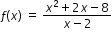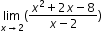# MA.912.C.1.1

Demonstrate understanding of the concept of a limit and estimate limits from graphs and tables of values.

### Examples

Example: For, estimateby calculating the function’s values for x=2.1, 2.01, 2.001 and for x=1.9, 1.99, 1.999. Explain your answer.
General Information
Subject Area: Mathematics (B.E.S.T.)
Strand: Calculus
Status: State Board Approved

## Related Courses

This benchmark is part of these courses.
1202300: Calculus Honors (Specifically in versions: 2014 - 2015, 2015 - 2022, 2022 and beyond (current))

## Related Access Points

Alternate version of this benchmark for students with significant cognitive disabilities.

## Related Resources

Vetted resources educators can use to teach the concepts and skills in this benchmark.

## Text Resource

No Limits For Usain:

This informational text resource is intended to support reading in the content area. The text uses the story of Usain Bolt and his quest to reduce his world record in the 100 meter race to raise the question of whether there is a limit to his—or any human's—athleticism. The article uses number series, limits, and convergent and divergent series to prove that, hypothetically at least, a world record will go on reducing beyond any limit. A logistic curve is shown to model the data.

Type: Text Resource

## Tutorials

Limit and Function Defined at Point of Discontinuity:

In this video we will determine if a limit exists at a point of discontinuity.

Type: Tutorial

Introduction to limits:

This video will provide an introduction to the intuition behind limits through graphing.

Type: Tutorial

Two-sided limit from a graph:

Determine the limit of a function from the left and from the right of an x-value from graph.

Type: Tutorial

## Student Resources

Vetted resources students can use to learn the concepts and skills in this benchmark.

## Tutorials

Limit and Function Defined at Point of Discontinuity:

In this video we will determine if a limit exists at a point of discontinuity.

Type: Tutorial

Introduction to limits:

This video will provide an introduction to the intuition behind limits through graphing.

Type: Tutorial

Two-sided limit from a graph:

Determine the limit of a function from the left and from the right of an x-value from graph.

Type: Tutorial

## Parent Resources

Vetted resources caregivers can use to help students learn the concepts and skills in this benchmark.